# [原创] 区块链量化投资系列课程(4)

### NO.1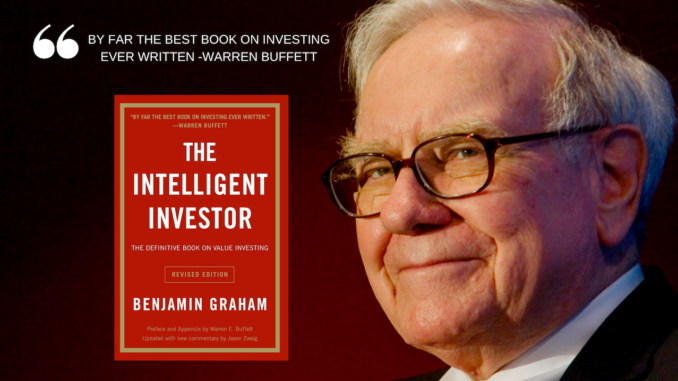• 把手中 50% 的资金投资于股票基金，剩下 50% 投资于债券基金。即股票和债券两者各占一半。

• 根据固定间隔时间或市场变化进行一次资产再平衡，使股票资产和债券资产的比例恢复到初始的 1:1。

### NO.2

• 如果，股票价格上涨，会使得股票的市值大于债券的市值，当两者市值比率超过设定的阈值时，则对总仓位进行重新调整，卖出股票，并且买入债券，使股债市值比例恢复至初始的 1:1。

• 反之，股票价格下跌，会使得股票的市值小于债券的市值，当两者市值比率超过设定的阈值时，则对总仓位进行重新调整，买入股票，并且卖出债券，使股债市值比例恢复至初始的 1:1。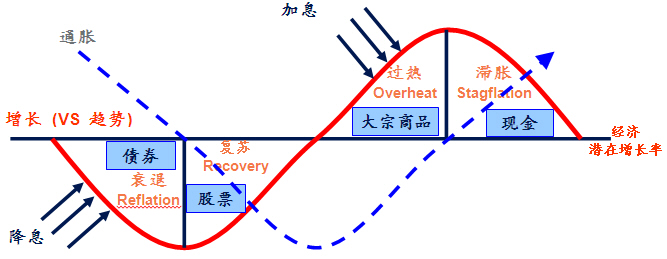### NO.3

• 按照当前的 BTC 的价值，账户余额保留￥5000 现金和 0.1个 BTC，即现金和BTC 市值的初始比例是 1:1。

• 如果 BTC 的价格上涨至￥6000，即 BTC 市值大于账户余额，并且其之间的差超过设定的阈值，就卖掉(6000-5000)/6000/2个币。说明 BTC 升值了，把钱兑换回来。

• 如果 BTC 的价格下跌至￥4000，即 BTC市值小于账户余额，并且其之间的差超过设定的阈值，就买入(5000-4000)/4000/2个币。说明 BTC 贬值了，把 BTC 买回来。

### NO.4

``````// 策略参数
var threshold = 0.05; // 阈值
var LoopInterval = 60; // 轮询间隔(秒)
var MinStock = 0.001; // 最小交易量
var XPrecision = 4; // 量精度
var ZPrecision = 8; // 价格精度

// 撤单函数
function CancelPendingOrders() {

}

// 下单函数
function onTick() {

}

// 主函数
function main() {
// 过滤非重要信息
SetErrorFilter("GetRecords:|GetOrders:|GetDepth:|GetAccount|:Buy|Sell|timeout");
while (true) { // 轮询模式
if (onTick()) { // 执行 onTick 函数
CancelPendingOrders(); // 取消未成交的挂单
}
Sleep(LoopInterval * 1000); // 休眠
}
}``````

### NO.5

``````// 下单函数
function onTick() {
var acc = _C(exchange.GetAccount); // 获取账户信息
var ticker = _C(exchange.GetTicker); // 获取 Tick 数据
var spread = ticker.Sell - ticker.Buy; // 获取 Tick 数据的买卖价差
// 账户余额与当前持仓价值的差值的 0.5倍
var diffAsset = (acc.Balance - (acc.Stocks * ticker.Sell)) / 2;
var ratio = diffAsset / acc.Balance; // diffAsset / 账户余额
LogStatus('ratio:', ratio, _D()); // 打印 ratio和当前时间
if (Math.abs(ratio) < threshold) { // 如果 ratio的绝对值小于指定阈值
return false; // 返回 false
}
if (ratio > 0) { // 如果 ratio大于 0
var buyPrice = _N(ticker.Sell + spread, ZPrecision); // 计算下单价格
var buyAmount = _N(diffAsset / buyPrice, XPrecision); // 计算下单量
if (buyAmount < MinStock) { // 如果下单量小于最小交易量
return false; // 返回 false
}
exchange.Buy(buyPrice, buyAmount, diffAsset, ratio); // 买入下单
} else {
var sellPrice = _N(ticker.Buy - spread, ZPrecision); // 计算下单价格
var sellAmount = _N(-diffAsset / sellPrice, XPrecision); // 计算下单量
if (sellAmount < MinStock) { // 如果下单量小于最小交易量
return false; // 返回 false
}
exchange.Sell(sellPrice, sellAmount, diffAsset, ratio); // 卖出下单
}
return true; // 返回 true
}``````

• 获取账户信息。

• 获取 Tick 数据。

• 计算 Tick 数据买卖价差。

• 计算账户余额和 BTC 市值价差。

• 计算买卖条件、下单价格、下单量。

• 下单，并返回 true。

### NO.6

``````// 撤单函数
function CancelPendingOrders() {
Sleep(1000); // 休眠 1秒
var ret = false;
while (true) {
var orders = null;
// 持续获取未成交订单数组，如果返回异常，则继续获取
while (!(orders = exchange.GetOrders())) {
Sleep(1000); // 休眠 1秒
}
if (orders.length == 0) { // 如果订单数组为空
return ret; // 返回撤单状态
}
for (var j = 0; j < orders.length; j++) { // 遍历未成交订单数组
exchange.CancelOrder(orders[j].Id); // 依次取消未成交订单
ret = true;
if (j < (orders.length - 1)) {
Sleep(1000); // 休眠 1秒
}
}
}
}``````

• 撤单前先等待 1 秒，个别交易所，你懂的。

• 持续获取未成交订单数组，如果返回异常，则继续获取。

• 如果未成交订单数组为空，即时返回撤单状态。

• 如果有未成交的订单，则遍历整个数组，并依次根据订单号撤单。

### NO.7

``````// 回测环境
/*backtest
start: 2018-01-01 00:00:00
end: 2018-08-01 11:00:00
period: 1m
exchanges: [{"eid":"OKCoin_EN","currency":"BTC"}]
*/

// 撤单函数
function CancelPendingOrders() {
Sleep(1000); // 休眠 1秒
var ret = false;
while (true) {
var orders = null;
// 持续获取未成交订单数组，如果返回异常，则继续获取
while (!(orders = exchange.GetOrders())) {
Sleep(1000); // 休眠 1秒
}
if (orders.length == 0) { // 如果订单数组为空
return ret; // 返回撤单状态
}
for (var j = 0; j < orders.length; j++) { // 遍历未成交订单数组
exchange.CancelOrder(orders[j].Id); // 依次取消未成交订单
ret = true;
if (j < (orders.length - 1)) {
Sleep(1000); // 休眠 1秒
}
}
}
}

// 下单函数
function onTick() {
var acc = _C(exchange.GetAccount); // 获取账户信息
var ticker = _C(exchange.GetTicker); // 获取 Tick 数据
var spread = ticker.Sell - ticker.Buy; // 获取 Tick 数据的买卖价差
// 账户余额与当前持仓价值的差值的 0.5倍
var diffAsset = (acc.Balance - (acc.Stocks * ticker.Sell)) / 2;
var ratio = diffAsset / acc.Balance; // diffAsset / 账户余额
LogStatus('ratio:', ratio, _D()); // 打印 ratio和当前时间
if (Math.abs(ratio) < threshold) { // 如果 ratio的绝对值小于指定阈值
return false; // 返回 false
}
if (ratio > 0) { // 如果 ratio大于 0
var buyPrice = _N(ticker.Sell + spread, ZPrecision); // 计算下单价格
var buyAmount = _N(diffAsset / buyPrice, XPrecision); // 计算下单量
if (buyAmount < MinStock) { // 如果下单量小于最小交易量
return false; // 返回 false
}
exchange.Buy(buyPrice, buyAmount, diffAsset, ratio); // 买入下单
} else {
var sellPrice = _N(ticker.Buy - spread, ZPrecision); // 计算下单价格
var sellAmount = _N(-diffAsset / sellPrice, XPrecision); // 计算下单量
if (sellAmount < MinStock) { // 如果下单量小于最小交易量
return false; // 返回 false
}
exchange.Sell(sellPrice, sellAmount, diffAsset, ratio); // 卖出下单
}
return true; // 返回 true
}

// 主函数
function main() {
// 过滤非重要信息
SetErrorFilter("GetRecords:|GetOrders:|GetDepth:|GetAccount|:Buy|Sell|timeout");
while (true) { // 轮询模式
if (onTick()) { // 执行 onTick 函数
CancelPendingOrders(); // 取消未成交的挂单
Log(_C(exchange.GetAccount)); // 打印当前账户信息
}
Sleep(LoopInterval * 1000); // 休眠
}
}``````

### NO.8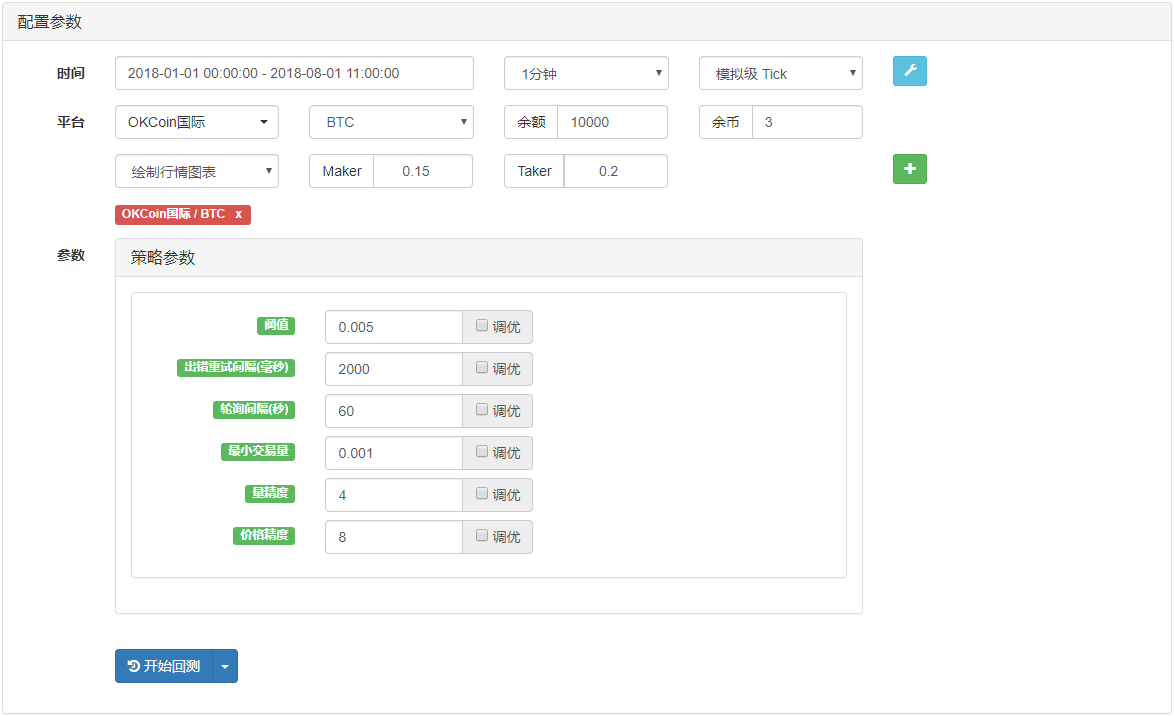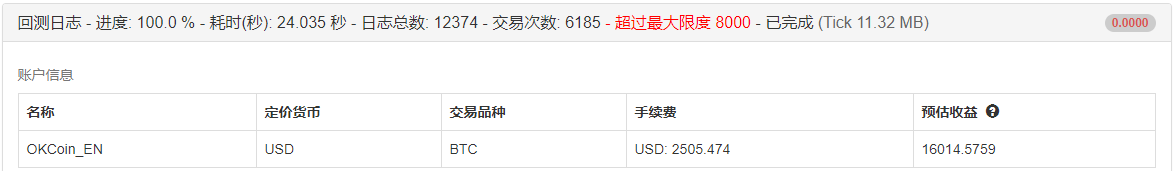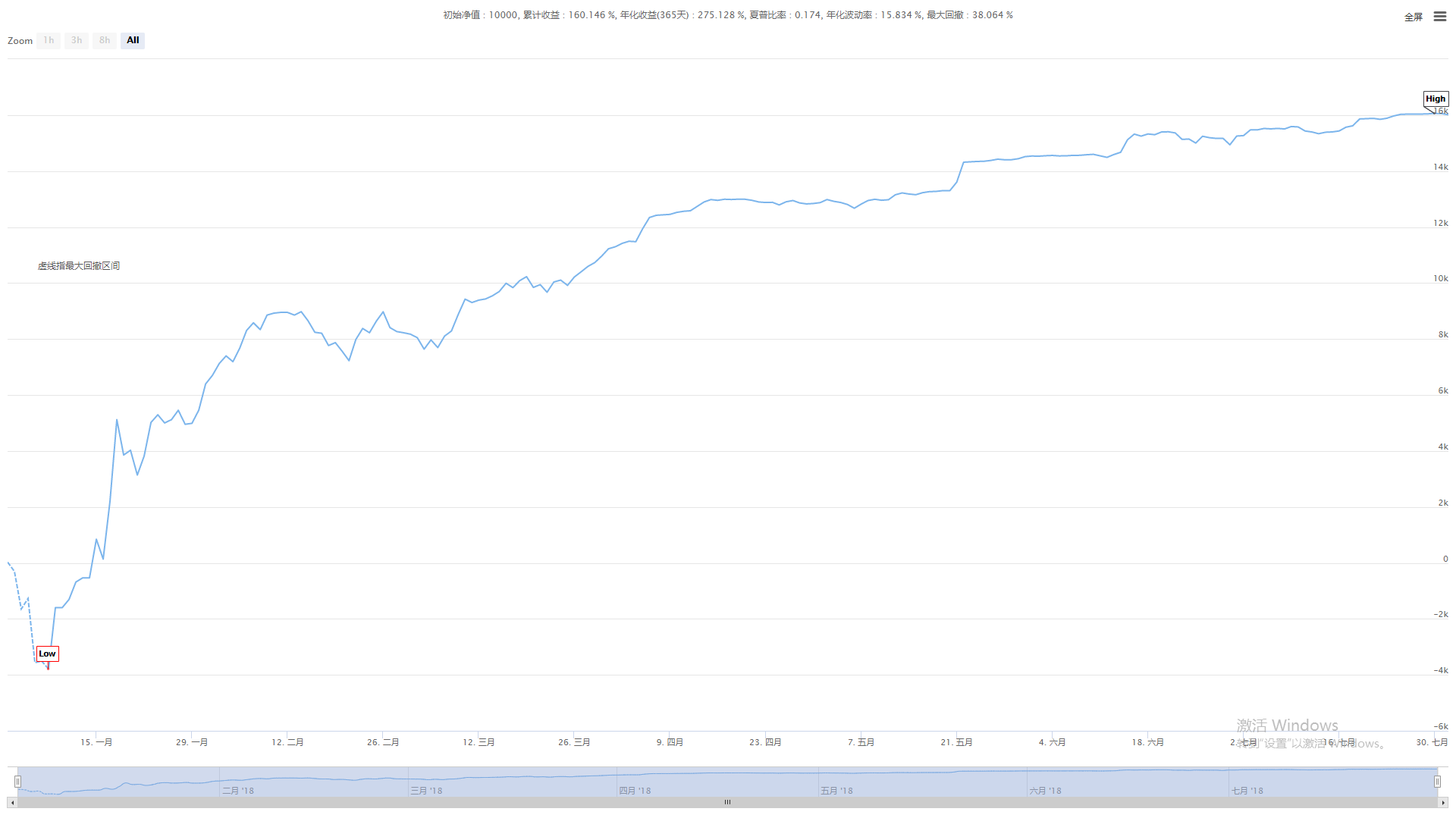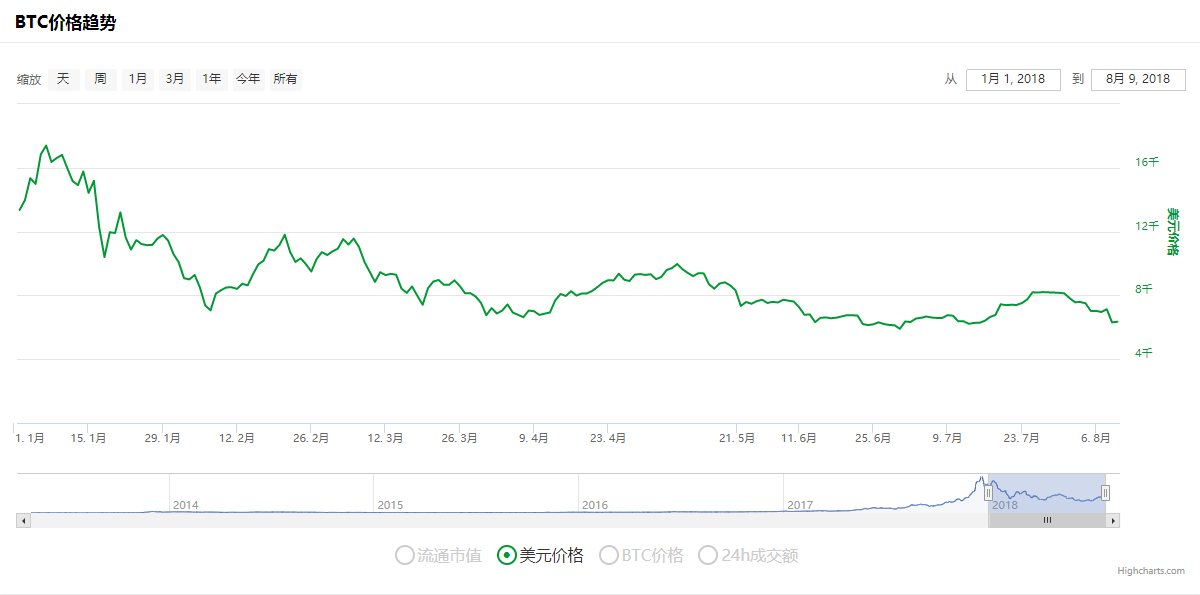BTC 已经持续了长达 8 个月下跌，甚至最大跌幅超过70%，这造成很多投资者对区块链资产失去信心。本篇策略累计收益高达 160%，年化收益风险比超过 5。对于一个这么简单的投资策略，这个投资回报率已经超过绝大多数梭哈的群众了。

### NO.9——本杰明 · 格雷厄姆《聪明的投资者》

##### 最新评论 (9)

• 2018-12-15 19:52:49

这个回测不是应该保证币和余额的价值相等么?

• 2018-12-08 15:02:37

这个策略6啊

• 2018-11-17 10:19:31

很棒的网站

• 2018-11-16 15:34:09

动态再平衡=反转策略

• 2018-11-16 15:22:12

动态平衡有个前提条件：就是进行价值估算

• 2018-11-16 15:34:44

👍

• 2018-11-16 15:13:15

做个聪明的投资者☺️

• 2018-11-14 11:19:58

赞，讲述明了

• 2018-11-14 11:10:09

喜欢❤️

Copyright © 2019 宽客在线